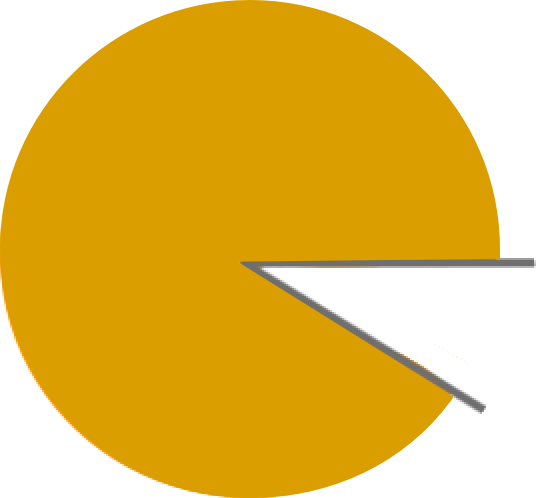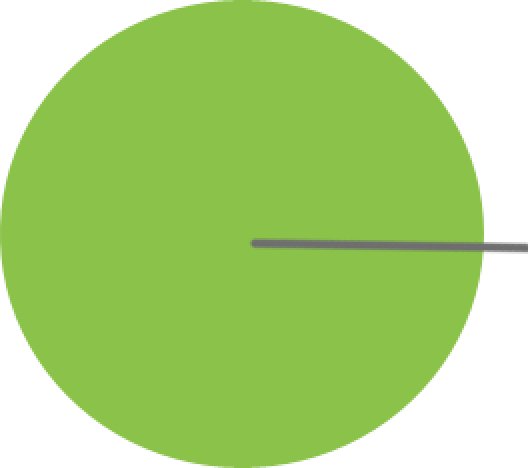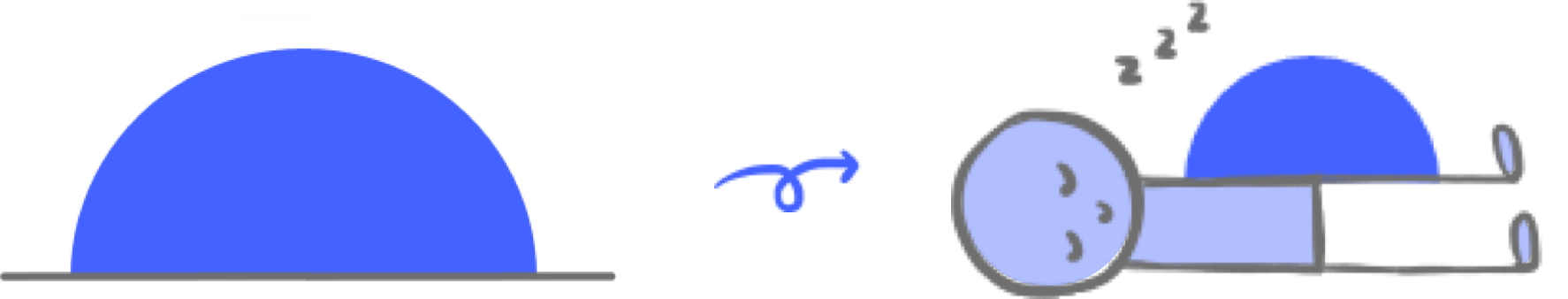•• Merch
• Donate
• SAT Prep

IntroductionIntroduction# Types of Angles

INTRO
An angle is a figure formed by two lines or rays connecting at a common point called a vertex.We identify angles based on the amount of space there is between the two lines. We measure this space in degrees (°) or radians - we'll be using degrees in this lesson.
There are six different types of angles based on size. To keep track of them, we imagine angles as stick figures in motion.
Pick an angle:An acute angle is an angle that measures less than 90°. We can imagine the angle leaning forward to see something cute.
Check out our
Calculator
or explore our
Lesson
and
Practice

CALCULATOR

## Identifying Angles Calculator

### Step 1. Describe the angle.

What do you know about the angle? Check at least one box ✅
 Looks like... The angle is...less than 90°equal to 90°greater than 90° and less than 180°equal to 180°greater than 180° and less than 360°equal to 360°
KEY STEPS

## How to Identify Angles

### Step 2. Identify the angleThe angle is:
• leaning forward to see something cute
• less than 90°
acute angleThe angle is:
• sitting upright
• equal to 90°
right angleThe angle is:
• leaning backward to work its abs
• greater than 90° and less than 180°
obtuse angleThe angle is:
• lying flat and straight
• equal to 180°
straight angleThe angle is:
• flexing and bending past a straight line
• greater than 180° and less than 360°
reflex angleThe angle is:
• rotated all the way around
• equal to 360°
full rotation angle
LESSON
Identifying Types of Angles

## Identifying Types of Angles

There are six different types of angles based on size, or the space between the two lines. Use the following flashcards to strengthen your memory of the different angle types.An angle that is less than 90° and looks like it's leaning forward to see something cute.
acute angle
✨ Click to flip! ✨
If you're not a fan of flashcards, continue with the lesson to see more examples another way!
PRACTICE
Identifying Types of Angles

## Practice: Identifying Types of Angles

Question 1 of 12:
What kind of angle is this?### Step 1. Describe the angle

The angle is...(check one box from each column)leaning forward to see something cute equal to 180°sitting upright equal to 90°leaning backward to work its abs greater than 180° and less than 360°lying flat and straight less than 90°flexing and bending past a straight line greater than 90° and less than 180°rotated all the way around equal to 360°

CONCLUSION
Incredible job, look at you go! Thanks for checking out this lesson ☺️🙏. Where to next?Leave Feedback